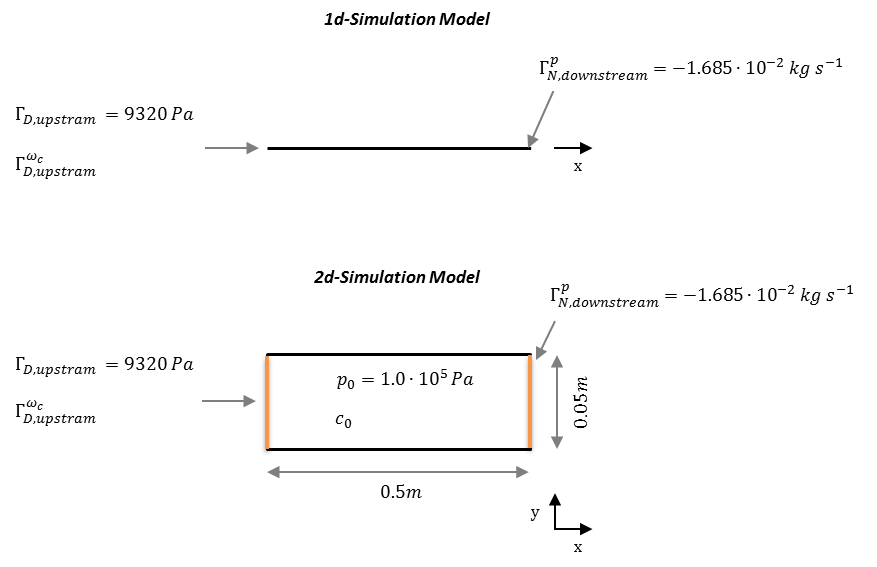## Overview

This scenario describes the transport of two solutes (Synthetica and Syntheticb) through a saturated media. Both solutes react to Productd according to $\text{Product d}=\text{Synthetic a}+0.5~\text{Synthetic b}$. The speed of the reaction is described with a first–order relationship $\frac{dc}{dt}=U(\frac{c_{\text{Synthetic a}}}{K_m+c_{\text{Synthetic b}}})$. The coupling of OGS-6 and IPhreeqc used for simulation requires to simulate the transport of $H^+$–ions, additionally. This is required to adjust the compulsory charge balance computation executed by Phreeqc. The solution by OGS-6–IPhreeqc will be compared to the solution by a coupling of OGS-5–IPhreeqc.

## Problem Description

1d scenario: The 1d–model domain is 0.5 m long and discretised into 200 line elements. The domain is saturated at start–up ($p(t=0)=$ 1.0e-5 Pa). A constant pressure is defined at the left side boundary ($g_{D,\text{upstream}}^p$) and a Neumann BC for the water mass out-flux at the right side ($g_{N,\text{downstream}}^p$). Both solutes, Synthetic a and Synthetic b are present at simulation start–up at concentrations of $c_{\text{Synthetic a}}(t=0)=c_{\text{Synthetic b}}(t=0)= 0.5~\textrm{mol kg}^{-1}~\textrm{water}$, the influent concentration is $0.5~\textrm{mol kg}^{-1}~\textrm{water}$ as well. Product d is not present at start–up ($c_{\text{Productd}}(t=0)=0$); neither in the influent. The initial concentration of $\text{H}^+$–ions is $1.0e\textrm{-}7~\textrm{mol kg}^{-1}~\textrm{water}$; the concentration at the influent point is the same. Respective material properties, initial and boundary conditions are listed in the tables below.

2d scenario: The 2d–scenario only differs in the domain geometry and assignment of the boundary conditions. The horizontal domain is 0.5 m in x and 0.5 m in y direction, and, discretised into 10374 quadratic elemtents with an edge size of 0.0025 m.Parameter Description Value Unit
$\phi$ Porosity 1.0
$\kappa$ Permeability 1.157e-12 $\textrm{m}^2$
$S$ Storage 0.0
$a_L$ long. Dispersion length 0.0 m
$a_T$ transv. Dispersion length 0.0 m
$\rho_w$ Fluid density 1.0e+3 $\textrm{kg m}^{-3}$
$\mu_w$ Fluid viscosity 1.0e-3 Pa s
$D_{\text{H}^+}$ Diffusion coef. for $\text{H}^+$ 1.0e-7 m$^2$ s
$D_{solutes}$ Diffusion coef. for Synthetica, Syntheticb and Productd 1.0e-12 m$^2$ s
$U$ Reaction speed constant 1.0e-3 h$^{-1}$
$K_m$ Half–saturation constant 10 mol kg$^{-1}$ water

Table: Media, material and component properties

Parameter Description Value Unit
$p(t=0)$ Initial pressure 1.0e+5 Pa
$g_{N,downstream}^p$ Water outflow mass flux -1.685e-02 mol kg$^{-1}$ water
$g_{D,upstream}^p$ Pressure at inlet 1.0e+5 Pa
$c_{Synthetica}(t=0)$ Initial concentration of Synthetica 0.5 mol kg$^{-1}$ water
$c_{Syntheticb}(t=0)$ Initial concentration of Syntheticb 0.5 mol kg$^{-1}$ water
$c_{Productd}(t=0)$ Initial concentration of Productd 0 mol kg${^-1}$ water
$c_{\text{H}^+}(t=0)$ Initial concentration of $\text{H}^+$ 1.0e-7 mol kg$^{-1}$ water
$g_{D,upstream}^{Synthetica_c}$ Concentration of Synthetica 0.5 mol kg$^{-1}$ water
$g_{D,upstream}^{Syntheticb_c}$ Concentration of Syntheticb 0.5 mol kg$^{-1}$ water
$g_{D,upstream}^{Productd}$ Concentration of Productd 0.0 mol kg$^{-1}$ water
$g_{D,upstream}^{\text{H}^+}$ Concentration of $\text{H}^+$ 1.0e-7 mol kg$^{-1}$ water

Table: Initial and boundary conditions

## Results

The kinetic reaction results in the expected decline of the concentration of Synthetic a and Synthetic b, which is super-positioned by the influx of these two educts through the left side. By contrast, the concentration of Product d increases in the domain. Over time, opposed concentration fronts for educts and Product d evolve. Both, OGS-6 and OGS-5 simulations yield the same results in the 1d as well as 2d scenario. For instance, the difference between the OGS-6 and the OGS-5 computation for the concentration of Product d expressed as root mean squared error is 1.76e-7 mol kg$^{-1}$ water (over all time steps and mesh nodes, 1d scenario); the corresponding median absolute error is 1.0e-7 mol kg$^{-1}$ water. This verifies the implementation of OGS-6–IPhreeqc.